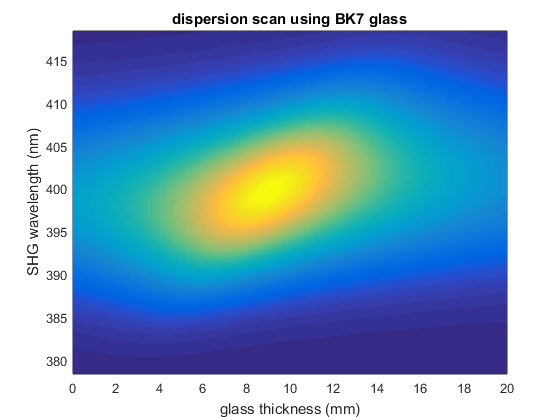# Example simulation of dispersion scan

Dispersion scan (D-scan) is a pulse compression technique related to chirp-scan . A sequence of second harmonic spectra is recorded, while introducing chirp by means of a glass wedge pair. A bidimensional map is thus optained, with frequency on one axis and thickness of glass on the other axis. The map is then fitted in order to extract the phase of the original pulse. This following script shows only the simulation of the D-scan map, not the fitting procedure.

 M. Miranda et al, Opt. Express 20, 688 (2012).

## Initialize laser pulse

```p = gaussianPulse('f0', 300/800, 't0', 0, 'fwhm', 20, ...
'dt',1, 'nPoints', 2^12);
p.polynomialPhase([2000 100 0 0])
```

## Define an optical medium

```p.medium = 'BK7';
```

## Precompensate the GDD

Experimentally this can be done using chirped mirrors. It is necessary because glass can only contribute positive GDD.

```p.groupDelayDispersion = p.groupDelayDispersion - 500;
```

## Introduce glass in the setup

```% array with glass thickness, along second dimension
glassThickness = linspace(0, 20, 100); % mm
% create a sequence of sub-pulse to hold the scan
p.propagate(glassThickness, 'mm');
```

## Calculate the second harmonic

```shg = p.harmonic(2);

figure()